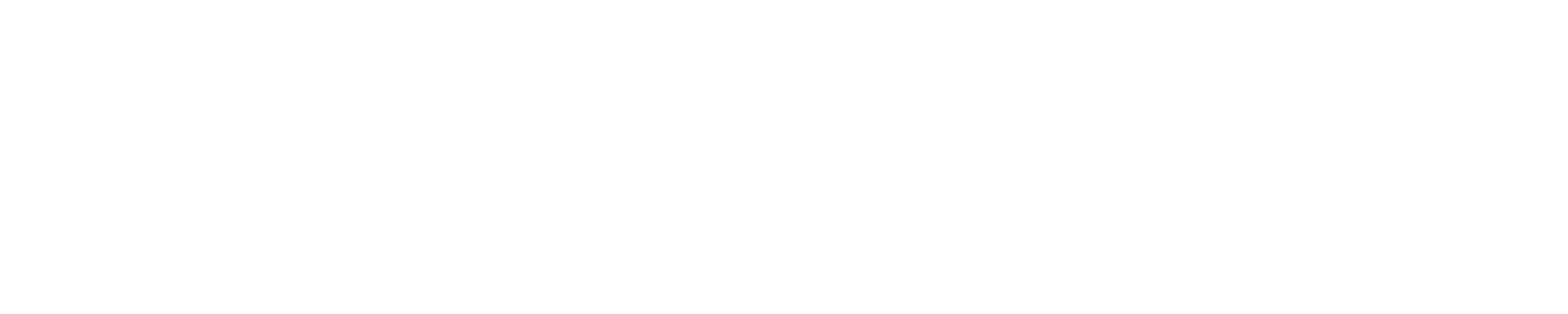# Capturing a Z-Wave byte value in HA

I’m trying to understand how Z-Wave works in HA in order to make modifications to an existing device.

The problem is as follows:

I have a Zipato Z-Wave alarm panel (see here for specific thread on improvements).

It sends an Alarm Report (version 2) described by Open Zwave here.

The alarm report also contains a user code which I would like to capture in HA. This states who changed the alarm state.

In the OZW log I can see this value (index 16 in the message so `0x01`):

``````Node013,   Received: 0x01, 0x10, 0x00, 0x04, 0x00, 0x0d, 0x0a, 0x71, 0x05, 0x00, 0x00, 0x00, 0xff, 0x06, 0x06, 0x01, 0x01, 0x67
2017-05-13 16:24:54.807 Detail,
2017-05-13 16:24:54.814 Info, Node013, Received Alarm report: type=0, level=0, sensorSrcID=0, type:Access Control event:6, status=255
``````

I know the `Info` line comes from here in the open Open Z-Wave code.

How would I go about capturing this value in HA?

My first attempt to add this value to the command class(the last value below) in the zwcfgXXXXXXXX.xml does not work:

``````<CommandClass id="113" name="COMMAND_CLASS_ALARM" version="2" request_flags="2" getsupported="false" innif="true">
<Instance index="1" />
<Value type="byte" genre="user" instance="1" index="0" label="Alarm Type" units="" read_only="true" write_only="false" verify_changes="false" poll_intensity="0" min="0" max="255" value="0" />
<Value type="byte" genre="user" instance="1" index="1" label="Alarm Level" units="" read_only="true" write_only="false" verify_changes="false" poll_intensity="0" min="0" max="255" value="0" />
<Value type="byte" genre="user" instance="1" index="2" label="SourceNodeId" units="" read_only="true" write_only="false" verify_changes="false" poll_intensity="0" min="0" max="255" value="0" />
<Value type="byte" genre="user" instance="1" index="9" label="Access Control" units="" read_only="true" write_only="false" verify_changes="false" poll_intensity="0" min="0" max="255" value="6" />
<Value type="byte" genre="user" instance="1" index="10" label="Burglar" units="" read_only="true" write_only="false" verify_changes="false" poll_intensity="0" min="0" max="255" value="3" />
<Value type="byte" genre="user" instance="1" index="16" label="User Code" units="" read_only="true" write_only="false" verify_changes="false" poll_intensity="0" min="0" max="255" value="3" />
</CommandClass>
``````

This adds the value in HA as a sensor but does it is not updated. So I guess I need to go deeper!

As I understand, there is 4 main components:

1. HA itself
2. Zwave config file (zwcfgXXXXXX.xml)
3. Python Open Z-Wave
4. Open-Wave

I am not sure if it is best to either create a new component, modify Z-wave config, modify open z-wave, or some combination of the aforementioned.

Any advice on the order I should go about doing this would be much appreciated.